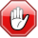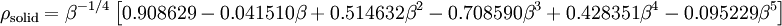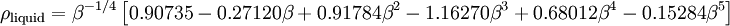# Lennard-Jones equation of stateThis page contains numerical values and/or equations. If you intend to use ANY of the numbers or equations found in SklogWiki in any way, you MUST take them from the original published article or book, and cite the relevant source accordingly.

The equation of state of the Lennard-Jones model.

## Equation of state

Johnson et al  propose an equation of state based on 33 parameters, which accurately reproduces the vapor liquid equilibrium curve.

## Melting line

The solid and liquid densities along the melting line are given by the equations of Mastny and de Pablo (Ref. 5 Eqs. 20 and 21):$\rho_{\mathrm {solid}} = \beta^{-1/4} \left[ 0.908629 - 0.041510 \beta + 0.514632 \beta^2 -0.708590\beta^3 + 0.428351 \beta^4 -0.095229 \beta^5\right]$

and$\rho_{\mathrm {liquid}} = \beta^{-1/4} \left[ 0.90735 - 0.27120 \beta + 0.91784 \beta^2 -1.16270\beta^3 + 0.68012 \beta^4 -0.15284 \beta^5\right]$